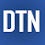## Pages

### Understanding Dropout Regularization in Neural Networks with Keras in Python

Dropout is a regularization technique to prevent overfitting in a neural network model training. The method randomly drops out or ignores a certain number of neurons in the network. Dropout technique is useful when we train two-dimensional convolutional neural networks to reduce overfitting with huge numbers of nodes in a network. Dropout can be added after the one or multiple layers in a neural network.

In this post, we'll briefly learn how to use dropout in neural network models with Keras in Python and its effect in model accuracy. The tutorial covers:
1. How to use Dropout layer in Keras model
2. Dropout impact on a Regression problem
3. Dropout impact on a Classification problem.

How to use Dropout layer in Keras model

To apply a dropout in Keras model, first, we load the Dropout class from the kares.layers module.

`from keras.layers import Dropout`

Then, we can add it to the multiple positions of the sequential model.
1. After the input layer

`model = Sequential()`
`model.add(Dense(16, input_dim=4, activation="relu")) `
`model.add(Dropout(0.2))`
`... `

2. Between the hidden layers

```model = Sequential()
...
`...`

3. Before the output layer

`model = Sequential() `
`...`
`model.add(Dropout(0.2))`
`model.add(Dense(3, activation="softmax"))`
`...`

The percent of nodes (neurons) to drop out is defined with the number. The value of 0.2 means 20 percent.

Dropout impact on a regression problem

We'll check the dropout effect on model accuracy by setting up multiple values. We'll create a simple regression model for Boston housing dataset and evaluate the model. Below is a full source code listing.

```from sklearn.datasets import load_boston
from keras.models import Sequential
from keras.layers import Dense
from keras.layers import Dropout
import matplotlib.pyplot as plt

x, y = boston.data, boston.target

dropouts = [0, 0.1, 0.2, 0.3, 0.4, 0.5, 0.6]
mses=[]
for d in dropouts:
model = Sequential()
```                        activation="relu"))
model.fit(x, y, epochs=30, batch_size=16, verbose=2)
l = model.evaluate(x, y)
mses.append(l)

plt.plot(dropouts, mses)
plt.ylabel("MSE")
plt.xlabel("dropout value")
plt.show()```

By increasing the dropout value, the MSE increases. Thus, we can not use higher values here.

Dropout impact on a classification problem

Next, we'll apply the same technique for classification problem with iris dataset. The same thing applies here too. The higher dropout value effects negatively to the accuracy level. Below is a full source code listing.

```from sklearn.datasets import load_iris
from keras.models import Sequential
from keras.layers import Dense
from keras.layers import Dropout
import matplotlib.pyplot as plt

x, y = iris.data, iris.target

accs=[]
for d in dropouts:
model = Sequential()
model.compile(loss="sparse_categorical_crossentropy", ```
```                      optimizer="adam", metrics=["accuracy"])
model.fit(x, y, epochs=40, batch_size=16, verbose=2)
acc = model.evaluate(x, y)
accs.append(acc[1:2])

plt.plot(dropouts,accs)
plt.ylabel("Accuracy")
plt.xlabel("dropout value")
plt.show()```

In this post, we've briefly learned how to use Dropout in a neural network model with Keras. Dropout is a regularization technique in model training. Commonly used value for dropout is 0.2 ( 20% percent).

1.Hello, I tried to run the code.
Everytime I have a different picture. I'm wondering why. Thanks.

from keras.models import Sequential
from keras.layers import Dense
from keras.layers import Dropout
import matplotlib.pyplot as grafico
%matplotlib inline
import tensorflow as tf

x, y = iris.data, iris.target

dropouts = [0, 0.1, 0.2, 0.3, 0.4, 0.5, 0.6]
accs=[]
for drop in dropouts:
modello = tf.keras.models.Sequential()
1.